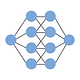# Intuitive Deep Learning Part 1a: Introduction to Neural Networks

## What is Deep Learning? A very gentle and intuitive introduction to Neural Networks and how they work!

Deep Learning has received a lot of hype in recent years due to its impressive performance on many applications, including language translation, medical diagnosis from X-rays, recognizing images to help with self-driving cars, beating the top Go players as well as beating high-ranking DotA players, learning how to play Atari games just from the pixel data… all these to name a few of Deep Learning’s recent accomplishments! In this post, we will (gently) introduce you to the inner workings behind Deep Learning at an intuitive level.

Deep Learning is really just a subset of Machine Learning that has garnered significant attention recently due to its stellar performance across many tasks as we’ve listed above.

That begs the question — What is Machine Learning? And how is Machine Learning any different from “traditional algorithms”?

If you’ve taken a class on algorithms, the standard metaphor for an algorithm is a recipe. An algorithm is a series of steps, that when performed in a specific order, produced your desired output. A “cooking algorithm” for a robot to make bread might go something like this:

And so on… (I’m not a bread expert, so I shan’t write a recipe for bread here)

In Machine Learning, we don’t specify the algorithm the way we did above. Rather, we specify the template (or the “architecture”) on what shape the cooking recipe might take:

The blanks are numbers that are not specified in the beginning but we will have to find out. We then write an algorithm (another series of instructions) to figure out those numbers according to what is “best” (we’ll define this later), relying on the data that we have access to.

At a very high level, the task of Machine Learning is thus two-fold:

A successful Machine Learning model needs both steps — without Step 1, it’s impossible to represent the right “recipe” without bread flour no matter how many times you try it; without Step 2, your proportions for your bread “recipe” might be completely wrong even though the ingredients are correct.

To give a more concrete example, consider the task of predicting house prices based on features such as house size (in sq m), number of stories, distance to nearest school (in m) etc.

A standard algorithm might perhaps be something like this: The house price is approximately (100 * house size) + (1000 * number of stories) — (30 * distance to nearest school. A (parametric) Machine Learning approach would look something like this:

Step 1: I’ve specified the template: The house price is __ * house size + __ * number of stories + __ * distance to nearest school.

Step 2: Looking at the data of all the houses I have listed, it seems that the best numbers to fill in the blanks is (90.3, 1006.2, -40.5) respectively.

We’ll dive deeper into exactly what templates we specify and how we can use the data to fill in those blanks. But at a high level, many Machine Learning tasks take a similar form to the example above: given some input, learn some function that transforms the input into a desired output.

Other examples of such input-output pairs are:

The power of Machine Learning algorithms is that we can solve problems we did not previously know the answer to. Suppose we didn’t know the formula for how to convert speech to text due to the sheer complexity of such a formula (if it even exists). With Machine Learning, we can figure that complex formula out just from data of transcription services (e.g. subtitles), solving (at least approximately) a problem we could not previously code the algorithm to.

Summary: Machine Learning consists of two steps: specify a template and find the best parameters for that template.

As mentioned above, Deep Learning is simply a subset of the architectures (or templates) that employs “neural networks” which we can specify during Step 1. “Neural networks” (more specifically, artificial neural networks) are loosely based on how our human brain works, and the basic unit of a neural network is a neuron.

At the basic level, a neuron does two things:

In (1), we often take some linear combination of the inputs. In layman terms, if we had three inputs to the neuron (let’s call them x1, x2, and x3), then we would combine them like this:

where the individual blanks are parameters to be optimized for later (i.e. learn from the data what numbers best fill in those blanks). In mathematical terms, the blanks that are attached to the inputs (x1, x2 and x3) are called weightsand the blank that is not attached to any input is called the bias.

With the linear combination, we apply some function (called the activation function) to achieve our eventual output. Common examples of these functions are:

That’s all there is to a neuron!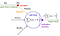Image taken from CS231N notes (http://cs231n.github.io/neural-networks-1/). This shows the parallels between the artificial neuron we’ve described and a biological neuron.

Now that we’ve described a neuron, a “neural network” is simply made out of layers of neurons, connected in a way that the input of one layer of neuron is the output of the previous layer of neurons (after activation):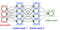Image taken from CS231N Notes (http://cs231n.github.io/neural-networks-1/). A layer in the neural network consists of one or more neurons. These layers are connected such that the input of one layer of neuron is the output of the previous layer of neurons (after activation).

Now, what’s the point of an activation function? The first step of the neuron makes sense, but is the second step really necessary? An activation function provides some non-linearity to the function, which we need to represent complex functions. See Footnote 1 for an explanation on why that is.

Let’s go through a simple example of a neural network to really solidify our understanding. Let’s say we’ve done our training (more on that later) and we’ve filled in the numbers to the template, so we’ve got a full model. Suppose we wish to predict whether someone will pass or fail their driving test. We have as input three features: age, sex, no. of lessons. We have two neurons in our intermediate layer. Let’s say that each neuron describes how well they will do in their parking and road driving respectively.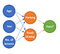Our simple neural network to predict whether someone has passed his/her driving

Now we’ve already filled in the best numbers, so we know the best way to describe someone’s performance on parking depending on their age, sex, and number of lessons. Let’s say the function is like this:A function to compute how well someone would park based on their age, sex and number of lessons

We consider two people:

Person A: A 20 year old, male, who has taken 10 lessons. The ‘parking’ neuron for him would output 0 (since ReLU converts all negative outputs to 0).

Person B: A 50 year old, female, who has taken 20 lessons. The ‘parking’ neuron for her would output 150.5 (since ReLU leaves positive outputs alone).

Similarly, for the road driving neuron, we could have a function like this:A function to compute how well someone would drive on the road based on their age, sex and number of lessons

For the above two people, we have Person A having a ‘road driving score’ of 0 and Person B has a ‘road driving score’ of 179.5.

Now, these two scores contribute to the final output of whether someone passed in this formula:A function to compute the probability of someone passing based on the output of the two intermediate neurons

At the final layer, we have calculated that Person A’s probability to pass would be Sigmoid(-3) = 4.74% and Person’s B probability to pass would be Sigmoid(2.9925) = 95.22%. Remember that sigmoid is a function to squeeze our value within 0 and 1, a function useful to get probabilities! You can find a sigmoid calculator here: https://keisan.casio.com/exec/system/15157249643325

At the end of the day, though, when the numbers have been filled into this template, neural networks are just complicated functions with neurons that build on other neurons. The power of neural networks is that in practice they turn out to have the flexibility to (approximately) represent well the underlying relationships between the task’s input and output.

As a side note: While the term neural network can refer to the template (model architecture), it is often also used to refer to the full model (with the parameters filled into the template).

There is an inaccuracy in our example that I would like to highlight. In our example, we knew exactly what the intermediate neuron was doing — calculating performance in parking and road driving. In neural networks, however, we have no idea what the neuron is doing; it figured out by itself what are the best intermediate features to compute that will lead to an accurate prediction of the final output. It could be ‘parking’ and ‘road driving’, or it could be something else we don’t really understand. That’s why people say neural networks are not explainable — we have no clue what those intermediate representations mean on a human-understandable level.

Let’s take another example. In recognizing whether an image contains a cat or not at the final layer, we might want intermediate layers to recognize intermediate features (e.g. the presence of certain cat-like features such as whiskers). Instead of hard-coding these intermediate features, we let the machine determine what intermediate features are best for recognizing cats. Whether this means that neurons in intermediate layers are recognizing whiskers exactly, we don’t know, but we trust that the machine knows how to arrange the parameters in the best possible form. We don’t specify those parameters, but we specify the architecture (the container) that sets boundaries for our models. We’ll talk more about this when we come to Part 2on Computer Vision.

And that’s a neural network! But as you might have noticed by now, there are many different combinations of how we can arrange this neural network architecture. How many hidden layers should we have? How many neurons should be in each hidden layer? All these settings that correspond to the architecture are called “hyper-parameters”, and we will eventually need to find the best set of “hyper-parameters” too. We’ll come back to this later in Part 1b.

Summary: A neural network is simply a complicated ‘template’ we specify which turns out to have the flexibility to model many complicated relationships between input and output.

Thus far, we’ve only touched on the “Step 1” of Machine Learning: setting in place a good template or architecture for our model. We now move on to “Step 2”: suppose we’ve found a good template, how do we learn from the data? Which set of numbers are “best” for our task? We call this optimization: finding the optimal (best) numbers that fit in our template.

To do so, we first have to define what “best” means with some kind of metric for how well the model performs. In cases where we are predicting some output (house prices, whether someone has cancer or not etc.), we want the prediction to be as close to the actual value as possible. If my prediction for the dataset is far off the actual value, then that’s really bad, and I might want to penalize that in my metric for performance.

The metric we use is known as the loss function, which describes how badly the model is performing based on how far off our predictions are from the actual value in our data-set. Our task is to come up with an algorithm to find the best parameters in minimizing this loss function.

One common loss function for a classification problem (see Footnote 2) is the softmax loss function (See Footnote 3).

Without giving the full details of the math, one common interpretation of this loss function is:

where Prob_model (CorrectLabel) refers to the probability that the model has assigned to what we know to be the correct label. Let’s go through an example to get a better intuition:

Suppose my problem is to identify whether an image is a hot dog or not:

Anyway, I give my model a training example of a hot dog (so we know “hot dog” is the correct label). Based on the image, my model might give me some probabilities like this:

Plugging this in the loss function for this training example, we have

Now take another model with a different set of parameters, where they try to predict from the same image. Instead, this model performs poorly in recognizing which is the correct image: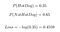Probabilities and loss for a poorer model which gives a lower probability to the correct class (Hot Dog)

Clearly, the model predictions are worse, and therefore we get a higher loss. Thus, the loss function does indeed correspond to how well the model is doing in predicting the correct class for a specific example. The higher the prediction on the correct answer, the lower the loss. The total loss can be seen as the average of the losses for each individual training example.

Now that we have a loss function, we have to find the right parameters to minimize it. For this, we turn to an algorithm called gradient descent. The idea behind gradient descent is simple — move the parameters slowly in the direction where the loss will decrease. The direction is given by the gradient of the loss with respect to those parameters. One “update step” thus looks like this:An update step: The new parameters is one step away from the old parameters. The step is in the direction of the gradient, and how big a step we take in that direction is determined by the step size.

After we’ve made our first step, we keep moving and iterating on these parameters until we’ve reached the ‘lowest point’, where no direction will give us any significantly lower loss:

You might often hear the term back-propagation. Back-propagation is merely a way to find the gradients in a neural network. We won’t go through the math here since finding gradients requires an understanding of calculus, but the idea is that a neural network is a really complicated function and taking derivatives the ‘traditional’ method might prove difficult. What the math shows, however, is that the gradient we need in an earlier layer can be expressed as a simpler function of the gradient in the layer after it:

What should we do then? A good strategy is to start at the very final layer, and work backwards. Suppose there are 5 layers: we find gradient of our final layer, layer 5(which is simple). Then, we find the gradient of layer 4, since we’ve already found the gradient of layer 5. Then, we find the gradient of layer 3, since we’ve found the gradient of layer 4, and so on until we reach layer 1. That’s why it’s called back-propagation: our calculations propagate backwards from layer 5 all the way to layer 1. Back-propagation really just helps us find somewhat simpler representations and formulas to calculate the gradients we need in order for gradient descent.

In case we’ve lost the big picture, let’s recap why we need to use gradient descent: Gradient descent helps us to find the parameters that minimize the loss. By minimizing training loss, we are getting a better model in terms of giving us the most accurate predictions for our training set.

Summary: Specifying a loss function and performing gradient descent helps us find the parameters that give the most accurate predictions for our training set.

Consolidated Summary: Machine Learning consists of two steps: specify a template and find the best parameters for that template. A neural network is simply a complicated ‘template’ we specify which turns out to have the flexibility to model many complicated relationships between input and output. Specifying a loss function and performing gradient descent helps us find the parameters that give the most accurate predictions for our training set.

What’s Next: So far, we’ve covered at a very high level the basic concept of Deep Learning.

Part 1b will go through the intuition of some other nuances we have to watch out for. We will go into some nitty-gritty details behind how to make Deep Learning models work, answering questions such as “How do we find the best model architecture (template)?”

This post comes with a coding companion if you are interested in coding your first neural network:

Footnotes:

If the output of each neuron was simply a linear combination of its inputs, then the output of the neuron in the layer output will still be a linear combination of the inputs, no matter how many hidden layers or how complex the neural network is. Suppose the contrary, that we didn’t have an activation function; then a neuron in hidden layer 2 is just a linear function of the outputs of neurons in hidden layer 1.

So the formulation of our neuron in hidden layer 2 might look something like this:

where h1, h2 and h3 are neurons in hidden layer 1. But the formulation of h1 would be

where x1 and x2 are the input features. The formulation of h2 and h3 will be similar, although the numbers filling the blanks might be different. Try substituting the formulation of h1, h2 and h3 in and you will realize the formulation of the neuron in hidden layer 2 can be simplified to a linear combination of the input features, as such: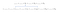Substituting in the formulation for neurons in earlier layers and rearranging it reveals that we merely get a linear function in the end

If you continue on with that logic, you will realize that even at the very last layer, they are simply linear combinations of the inputs — which really isn’t a very complex function to model complicated relationships. Adding a non-linearity means that you cannot rearrange it to get a linear function, and you end up with a pretty complicated formulation (to express complicated relationships):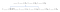Substituting in the formulation for neurons in earlier layers, we see that this representation does not reduce to a simple linear combination of the inputs

2. The difference between regression and classification

The output of supervised learning often falls into two categories: regression and classification. Classification is what this post focuses on: there are a few distinct outputs (called classes) that the input could be mapped to and there is a single correct label for each input. Regression, on the other hand, predicts a real-valued quantity rather than a class. Suppose we wish to predict Yelp star-ratings (1-star to 5-stars) from their comments. A classification approach predicts which distinct class (1-star, 2-stars, 3-stars, 4-stars or 5-stars) the review belongs to; a regression approach predicts some real-valued number (e.g. 3.71). For most of the posts in this series, we will focus on classification problems.

3. Softmax

Softmax is a common step at the end which converts the final outputs to a set of probabilities. Probabilities must fulfill the following criteria: each probability is between 0 and 1 (inclusive), and all probabilities sum up to 1.

If we do not have a softmax layer, there is no guarantee that our final outputs satisfy the conditions above, since the final output can be any number that won’t make any sense as probabilities (e.g. -4, 5.2 etc). Softmax thus normalizes all our final outputs to a set of probabilities to fulfill the conditions above.

Other Resources:

A good website to play with neural networks is TensorFlow Playground: https://playground.tensorflow.org

Written by

Written by

## Joseph Lee Wei En

#### I want to make Deep Learning concepts as intuitive and as easily understandable as possible by everyone! Follow my publication to learn more!